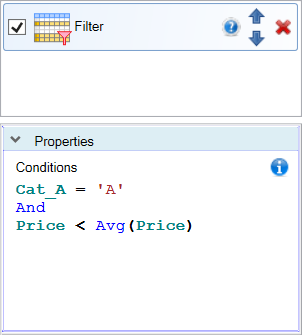# Reshape.XL Formula Expressions

Reshape.XL Expressions is a small tool - a language that significantly extends the capabilities of selected add-in functions, such as Filter, Summarize or Mutate. Expressions allow you to create more complex types of data analysis in a relatively simple way.

An example is the Filter function. Imagine that you need to filter out values from your table that are smaller than the average of the column. Or, you want to use the Mutate function to calculate the difference between the average and the current value of a variable in a row. With Reshape.XL Expressions, you can do these analyses very easily and quickly.## Syntax

Using Expressions within individual functions is described in the documentation. Here you find a list of the features that you can use in Expressions. Expressions consist of a function name, a table variable name, operators and directly written values.

Directly entered values must be expressed in invariant culture locale. This means, for numeric values you use a dot to separate decimal places and dates are in #YYYY-MM-DD# format, such as #2020-05-31#.

For example, an expression could be:
`PriceDiff = ( Price / Avg(Price) ) * VAT`
In this case, PriceDiff variable will contain the difference between the current value in the price variable and its average value across the column, multiplied by the value of the VAT variable. In general, expressions are simple equations with table variable names and built-in functions such as Avg, Min or Max.

To use a custom text string, you insert it into simple single quotation marks:
`LastName = 'John'`
Numeric values or variable names from the input table are not enclosed in quotation marks. Individual functions can be linked using various operators.

## Operators

Concatenation is allowed using Boolean AND, OR, and NOT operators. You can use parentheses to group clauses and force precedence. The AND operator has precedence over other operators. For example:
`(FirstName = 'John' OR FirstName= 'Jones') AND LastName = 'John'`
In addition you can use several comparison and arithmetic operators:
`<` `>` `<=` `>=` `<>` `IN` `LIKE` `+`        (addition)
`-`        (subtraction)
`*`        (multiplication)
`/`        (division)
`%`        (modulus)
`+`        (string concatenation)

## Wildcard Characters

Both the * and % can be used interchangeably for wildcard characters in a LIKE comparison. If the string in a LIKE clause contains a * or %, those characters should be enclosed in brackets ([ ]). If a bracket is in the clause, each bracket character should be enclosed in brackets (for example [ [ ] or [ ] ]). A wildcard is allowed at the start and end of a pattern, or at the end of a pattern, or at the start of a pattern. For example:
`ItemName LIKE '*water*'`       (contains water in the string)
`ItemName LIKE '*water'`        (ends with word water)
`ItemName LIKE 'water*'`        (starts with word water)
Wildcard characters are not allowed in the middle of a string. For example, 'te*xt' is not allowed.

## Aggregates

The following aggregate types are supported:
`Sum `        (Sum)
`Avg `        (Average)
`Min `        (Minimum)
`Max `        (Maximum)
`Count `    (Count)
`StDev `    (Statistical standard deviation)
`Var `        (Statistical variance)
In expressions you can combine aggregate functions and variables:
`Sum(Price) / Count(Price)` `Sum(Price / Avg(Discount))`

## Functions

Reshape.XL expressions includes several additional functions that you can use:

##### CONVERT(expression, type)

Converts particular expression to a specified .NET Framework Type. With expression
`Convert(Price, 'System.Int32')`
you can convert numerical variable to integer. As the type you can use Boolean, Byte, SByte, Int16, Int32, Int64, UInt16, UInt32, UInt64, String, Char, DateTime and TimeSpan.

##### LEN(expression)

Gets the length of a string. You can for example count the length of names in variable using the following expression:
`Len(FirstName)`

##### ISNULL(expression, replacement_value)

Checks an expression and either returns the checked expression or a replacement value. If expression is null, replacement_value is returned. Example gets -1 value if the cell in Price column is empty:
`IsNull(Price, -1)`

##### IIF(expresion, true_part, false_part)

Gets one of two values depending on the result of a logical expression. The following expression checks the price:
`IFF(Price > 1000, 'expensive', 'dear')`

##### TRIM(expression)

Removes all leading and trailing blank characters like \r, \n, \t and ' '

##### SUBSTRING(expression, start, length)

Gets a substring of a specified length, starting at a specified point in the string. Example:
`Substring(PhoneNumber, 7,8)`

Reshape.XL Expressions are simple to learn, easy to use, but in combination with high-level functions (such as Filter, Summarize or Mutate) they are very powerful in the case of complex data processing. Transformations, summarizations and mutating is with these expressions very useful. Individual practical use case you can find in the dokumentation for individual functions. Other interesting “hidden feature” that extends Reshape.XL functions are Regular Expressions. These are used for textual data processing and are described individually